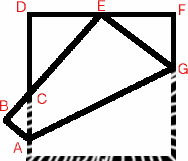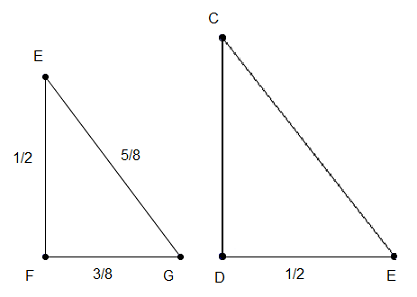#### You may also like### Fitting In

The largest square which fits into a circle is ABCD and EFGH is a square with G and H on the line CD and E and F on the circumference of the circle. Show that AB = 5EF. Similarly the largest equilateral triangle which fits into a circle is LMN and PQR is an equilateral triangle with P and Q on the line LM and R on the circumference of the circle. Show that LM = 3PQ### Look Before You Leap

Can you spot a cunning way to work out the missing length?### Triangle Midpoints

You are only given the three midpoints of the sides of a triangle. How can you construct the original triangle?

# Napkin

##### Age 14 to 16 Challenge Level:

This solution is a combination of the work of David from Trinity School and several others.

A square is folded so that the corner $E$ coincides with the midpoint of an opposite edge as shown in the diagram. The length of the edge of the square is 1 unit and the problem is to find the lengths and areas of the three triangles.The table shows the solutions:

 Triangle Lengths of sides 3 : 4 : 5 Ratio of lengths in 3 triangles Area Ratio of areas of 3 triangles EGF 3/8 : 1/2 : 5/8 3 3/32 9 CED 1/2 : 2/3 : 5/6 4 1/6 16 CAB 1/8 : 1/6 : 5/24 1 1/96 1

This is David's method:

The edge, $EF = 1/2$. Taking $FG = a$ then $GE = 1-a$

As it has a corner of the paper as part of it, we know $EGF$ is a right angled triangle, and so $GE^2 = EF^2 + FG^2$, which gives $(1-a)^2 = 1/4 + a^2$

so $a = 3/8.$

Now that we have the length of $a$, we have the lengths of all the sides of this triangle: $EF = 1/2$ , $FG = 3/8$ and $GE = 5/8.$

At this point, David uses Trigonometry to complete the problem, but other solutions just used similar triangles.

The area of triangle $EFG = 1/2$ base $\times$ height $= 3/32$.

Using this information and trigonometry, we can work out the angle $GEF$: $\angle GEF = \tan^{-1}(0.375 / 0.5) = \tan^{-1}0.75 = 36.9^{\circ}.$

taking the angle to the nearest tenth of a degree. Knowing this, we can work out the angle $DEC=53.1^{\circ}$ and use it with the length $DE$ to work out the length $DC=2/3.$ The last part needed of this triangle is $CE$ and, using Pythagoras' theorem, we get $CE =5/6.$

So $DE = {1 \over 2}$, $DC = {2 \over 3}$, $CE = {5 \over 6}$ and the area of triangle $DEC = 1/2 \times 2/3 \times 1/2 = {1 \over 6}.$

The last triangle needed is $ABC$. We know the length $BC = 1 - CE = 1/6.$ We can also work out the angle $BCA$ which is equal to angle $DCE$ and to angle $FEG.$ We can now use trigonometry to work out the length $AB = 1/6 \tan BCA = 1/8.$

And so once again, using Pythagoras, we can work out the length of line $AC$ which is $5/24.$

And so we have the final set of lengths: $AB = 1/8$, $BC = 1/ 6$ and $AC = 5/24.$

The area of triangle $ABC = 1/2 \times 1/8 \times 1/6 = 1/96$.

This means the total area of all the paper with a single thickness = $26/96 = .270833..$

This can be backed up by working out the area of the trapezium $ABEG$, subtracting the area of triangle $ABC$ and then multiplying the result by 2 to give the area of the original square that is now double thickness.

Area of trapezium = $1/2 [1/8 +5/8] \times 1 = 3/8.$ Double thickness area = $3/8 - 1/96 = 35/96.$

The total area is $2 \times 35/96 + 26/96= 1$ and so all areas are worked out and recorded, and the total area of single thickness paper and double thickness areas are recorded.

Here is the Similar Triangles method:Triangles EFG, CDE and ABC are similar triangles. This is how I know: firstly all three are right-angled triangles. Angle GEF and EGF add up to 90, as do angles CED and GEF. Therefore angles EGF and CED must be the same.This tells me that angle DCE must be the same as GEF, because triangle CDE is right-angled. Angle BCA and DCE are opposite angles so they must be equal, meaning that angle BAC is equal to CED. All three triangles have the same three angles, therefore they are similar.Triangle EFG has sides in the ratio $3:4:5$, so triangle CDE must also have sides in the ratio $3:4:5$.

$CD = {1 \over 2} \div 3 \times 4$

$CD = {2 \over 3}$

$CE = {1 \over 2} \div 4 \times 5$

$CE = {5 \over 8}$

$EB$ is a side of the square, so $EB$ is 1 unit.
$BC = 1- {5 \over 6}$
$BC = {1 \over 6}$

$BC$ is the $4$ side of the 3:4:5 triangle.

$BA = {1 \over 6} \div 4 \times 3$

$BA = {3 \over {24}}$

$AC = {1 \over 6} \div 4 \times 5$

$AC = {5 \over {24}}$